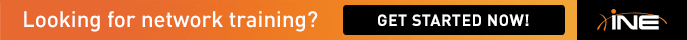# ASA Threat Detection

I am confused as to how the burst rate is calculated. If we take the following formula in input the values from the example it comes up with a different number than is given in the solution.

max(1/30 *<rate_interval>, 10)

max(1/30 * 7200) which give me 240 seconds

Now the only way i was able to make the math come out is if I did the following.

240 second / 10 seconds = 24 seconds

Then

24 seconds * 1000 packets per second = 24,000 packets per second.

Is the calculation correct? Can you explain why we caculate it this way?

Also the following is stated "The burst-rate interval is calculated automatically with the formula above." in the writeup. They way I read this I shouldn't have to input the burst-rate however the command is not accepted without that value. Can you explain further what you meant by this?

• Hi,

So you ended up with 240 seconds, which is correct. As the task states to acomodate for 1000 drops in 10 seconds, what's the value for 240 seconds? x=(240*1000)/10=24.000. The burst-rate value is manually configured, the burst-rate interval is automatically configured. Here's a very good document for further reading.

http://www.cisco.com/c/en/us/support/docs/security/asa-5500-x-series-next-generation-firewalls/113685-asa-threat-detection.html

Regards,
Cristian.

•

Thanks for the link it was helpful but I am still a little confused. I get the math now but i still don't understand the relationship between average and burst. The formual is max(1/30th * average_rate, 10) = X seconds. What is this X seconds? Is it the BRI?

After I have the BRI I need to calculate the BR. I do this with another forumla that isn't explained anywhere (BRI * Provided burst drops? )/ Number of seconds takes to reach provided burst value.

So it seems you will always be provided or need to decide

1. What is my average rate?
2. What is my average rate interval?
3. What are the number of drops I consider a burst?
4. Over how many seconds does my burst value accumulate?

Using this I will create my own example and let me know if it makes sense.

1. Average Rate = 5,000 drops
2. Average Rate Interval = 1 hour 3600 seconds
3. Number of packets I consider a burst. = 700 drops
4. Number of seconds to accumulate the burst = 15 seconds

max(1/30 * ARI, 10) = BRI

max(1/30 * 3600, 10) = BRI

max(1/30 * 3600, 10 ) = 120 ; BRI = 120

So based on my average rate and average rate interval I need to calculate the burst rate.

(BRI * number of packets I considers a burst)/ time I takes to accumulate the burst = BR

(120 * 700) /15 = BR

BR = 5,600 drops

My logic correct?

• Hi,

So average-rate value and average-rate interval are configurable, burst-rate value is configurable, but burst-rate interval is NOT. Yes, max(1/38 * ARI, 10) is the BRI. Yes your example is correct, basically you detect the BRI (120) with the formula and in order to accomodate for 700 drops (BR value) in 15 seconds (BRI), you detect the requested BR value (5600).

Regards,
Cristian.

• Ok cool I get it now. You are given the values and for the BR you input in the command you need to convert provide BR so that the AR and BR follow the same interval ratio. The second part wasn't explained well intially. Thanks for the clarifiation.

Eric